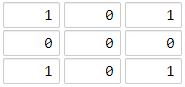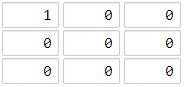1162. As Far from Land as Possible

Given an `n x n` `grid` containing only values `0` and `1`, where `0` represents water and `1` represents land, find a water cell such that its distance to the nearest land cell is maximized, and return the distance. If no land or water exists in the grid, return `-1`.

The distance used in this problem is the Manhattan distance: the distance between two cells `(x0, y0)` and `(x1, y1)` is `|x0 - x1| + |y0 - y1|`.

Example 1:```Input: grid = [[1,0,1],[0,0,0],[1,0,1]]
Output: 2
Explanation: The cell (1, 1) is as far as possible from all the land with distance 2.
```

Example 2:```Input: grid = [[1,0,0],[0,0,0],[0,0,0]]
Output: 4
Explanation: The cell (2, 2) is as far as possible from all the land with distance 4.
```

Constraints:

• `n == grid.length`
• `n == grid[i].length`
• `1 <= n <= 100`
• `grid[i][j]` is `0` or `1`

1162. As Far from Land as Possible
``````struct Solution;
use std::collections::VecDeque;

impl Solution {
fn max_distance(mut grid: Vec<Vec<i32>>) -> i32 {
let n = grid.len();
let m = grid.len();
let mut queue: VecDeque<(usize, usize, i32)> = VecDeque::new();
for i in 0..n {
for j in 0..m {
if grid[i][j] == 1 {
queue.push_back((i, j, 0));
}
}
}
let mut res = -1;
let offsets = [(1, 0), (0, 1), (-1, 0), (0, -1)];
while let Some((i, j, d)) = queue.pop_front() {
for offset in &offsets {
let i = i as i32 + offset.0;
let j = j as i32 + offset.1;
if i >= 0 && j >= 0 && i < n as i32 && j < m as i32 {
let i = i as usize;
let j = j as usize;
if grid[i][j] == 0 {
grid[i][j] = 1;
res = res.max(d + 1);
queue.push_back((i, j, d + 1));
}
}
}
}
res as i32
}
}

#[test]
fn test() {
let grid = vec_vec_i32![[1, 0, 1], [0, 0, 0], [1, 0, 1]];
let res = 2;
assert_eq!(Solution::max_distance(grid), res);
let grid = vec_vec_i32![[1, 0, 0], [0, 0, 0], [0, 0, 0]];
let res = 4;
assert_eq!(Solution::max_distance(grid), res);
}
``````